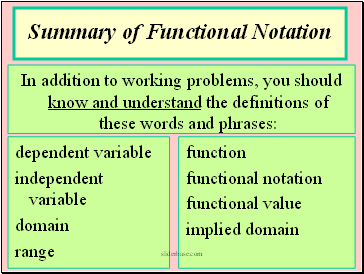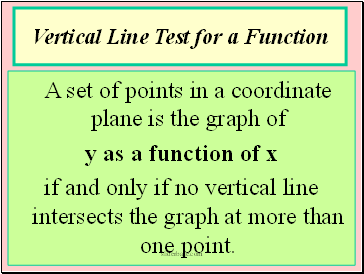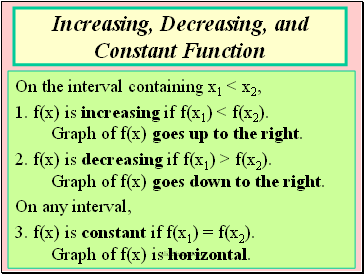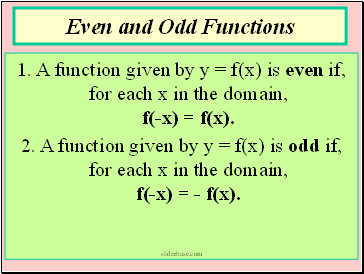# Functions and GraphsPage 2

#### WATCH ALL SLIDES

Generally, the domain is implied to be the set of all real numbers that yield a real number functional value (in the range).

Some restrictions to domain:

1. Denominator cannot equal zero (0).

2. Radicand must be greater than or equal to zero (0).

3. Practical problems may limit domain.

Slide 10## Summary of Functional Notation

dependent variable

independent variable

domain

range

function

functional notation

functional value

implied domain

In addition to working problems, you should know and understand the definitions of these words and phrases:

Slide 11## Vertical Line Test for a Function

A set of points in a coordinate plane is the graph of

y as a function of x

if and only if no vertical line intersects the graph at more than one point.

Slide 12On the interval containing x1 < x2,

1. f(x) is increasing if f(x1) < f(x2). Graph of f(x) goes up to the right.

2. f(x) is decreasing if f(x1) > f(x2). Graph of f(x) goes down to the right.

On any interval,

3. f(x) is constant if f(x1) = f(x2). Graph of f(x) is horizontal.

Increasing, Decreasing, and Constant Function

Slide 13## Even and Odd Functions

1. A function given by y = f(x) is even if, for each x in the domain, f(-x) = f(x).

2. A function given by y = f(x) is odd if, for each x in the domain, f(-x) = - f(x).

Go to page:
1  2